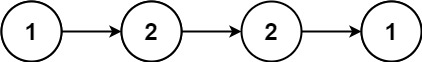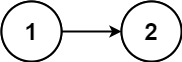# 234. Palindrome Linked List | LeetCode Solution

Given the `head` of a singly linked list, return `true` if it is a palindrome.

Example 1:```Input: head = [1,2,2,1]
Output: true
```

Example 2:```Input: head = [1,2]
Output: false
```

Constraints:

• The number of nodes in the list is in the range `[1, 105]`.
• `0 <= Node.val <= 9`

## The solution to the above question is-

/**
* struct ListNode {
*     int val;
*     ListNode *next;
*     ListNode() : val(0), next(nullptr) {}
*     ListNode(int x) : val(x), next(nullptr) {}
*     ListNode(int x, ListNode *next) : val(x), next(next) {}
* };
*/
class Solution
{
public:
{
ListNode *prev = NULL;
ListNode *next = NULL;
{
}
return prev;
}

{
return true;

while (fast->next && fast->next->next)
{
slow = slow->next;
fast = fast->next->next;
}
slow->next = reverse(slow->next);
slow = slow->next;
while (slow != NULL)
{